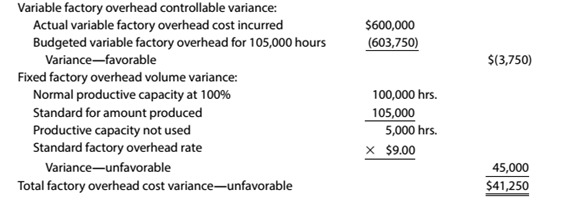Chapter 13, Problem 13.29E

Chapter
Section
Textbook Problem

Factory overhead variance correctionsThe data related to Danville Sporting Goods Company's factory overhead cost for the production of 40,000 units of product are as follows:Productive capacity at 100% of normal was 100.000 hours, and the factory overhead cost budgeted at the level of 105,000 standard hours was $720,000. Based on these data, the chief cost accountant prepared the following variance analysis:Identify the errors in the factory overhead cost variance analysis. To determine Concept Introduction: The variable factory overhead controlled variance is difference between the budgeted and actual variable factory overhead. The fixed factory overhead volume variance is the difference between the standard fixed factory overhead at actual production and at 100% of normal capacity. The sum of variableoverhead controlled variance and fixed factory overhead volume variance is thefactory overhead cost variance. The error in factory overhead cost variance analysis. Explanation Calculation of variable factory overhead controlled variance is as follows: Variable factory overhead controlled variance = ( Actual Variable factory overhead Budgeted Variable factory overhead for 105,000 hours variance)=[269,000(105,000×$5.75)]=269,000$603,750=$334,750 Favourable Variance

Now, the calculation of fixed factory overhead rate given below:

Fixed factory overhead rate =( Standard rate of factory overhead Standardrate of variable factory overhead)=($9$5.75)=\$3

Still sussing out bartleby?

Check out a sample textbook solution.

See a sample solution

The Solution to Your Study Problems

Bartleby provides explanations to thousands of textbook problems written by our experts, many with advanced degrees!

Get Started